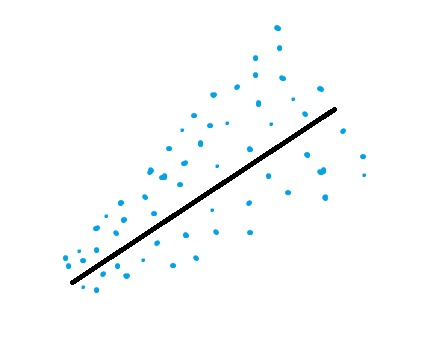# White Test: Definition, Examples

Share on

Statistics Definitions > White Test

## What is the White Test?

White’s test is used to test for heteroscedastic (“differently dispersed”) errors in regression analysis. It is a special case of the (simpler) Breusch-Pagan test.A graph showing heteroscedasticity; the White test is used to identify heteroscedastic errors in regression analysis.

The null hypothesis for White’s test is that the variances for the errors are equal. In math terms, that’s:
H0 = σ2i = σ2.
The alternate hypothesis (the one you’re testing), is that the variances are not equal:
H1 = σ2i ≠ σ2.

The only different between White’s test and the Breusch-Pagan is that its auxiliary regression doesn’t include cross-terms or the original squared variables. Other than that, the steps are exactly the same.

## When Should You Use White’s Test?

If your data set has many explanatory variables, the test may be challenging to calculate. Unless you have a specific reason for running the White Test (i.e. you need your independent variable to have a interactive, non linear effect on the variance), you should use the simpler Breusch-Pagan. White’s test is an asymptotic test, which is meant to be used on large samples. For smaller samples, interpret the results with caution.

One issue with White’s test us that it can return a significant result even if the variances of the errors are equal. This happens because the model is a general one, and may pick up other issues in your data (although it won’t specify what those issues are!). According to Richard Williams, one of the reasons the test is more general is because of added terms that test for more types of heteroscedasticity, like adding squares of regressors (i.e. the independent variables) to try and identify non-linear shapes like an hourglass.

## References

Kotz, S.; et al., eds. (2006), Encyclopedia of Statistical Sciences, Wiley.
White, H. (1980). “A Heteroskedasticity-Consistent Covariance Matrix Estimator and a Direct Test for Heteroskedasticity”. Econometrica. 48 (4): 817–838
Williams, R. Retrieved Jun 27, 2016 from: https://www3.nd.edu/~rwilliam/stats2/l25.pdf.

CITE THIS AS:
Stephanie Glen. "White Test: Definition, Examples" From StatisticsHowTo.com: Elementary Statistics for the rest of us! https://www.statisticshowto.com/white-test/
---------------------------------------------------------------------------Need help with a homework or test question? With Chegg Study, you can get step-by-step solutions to your questions from an expert in the field. Your first 30 minutes with a Chegg tutor is free!